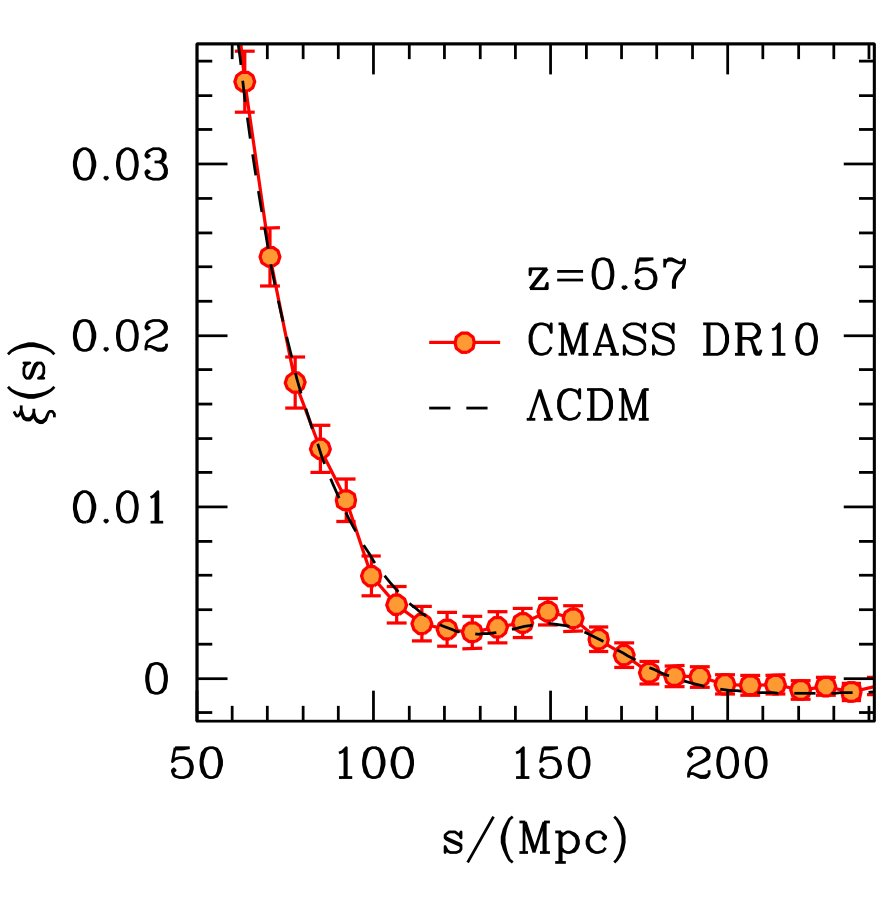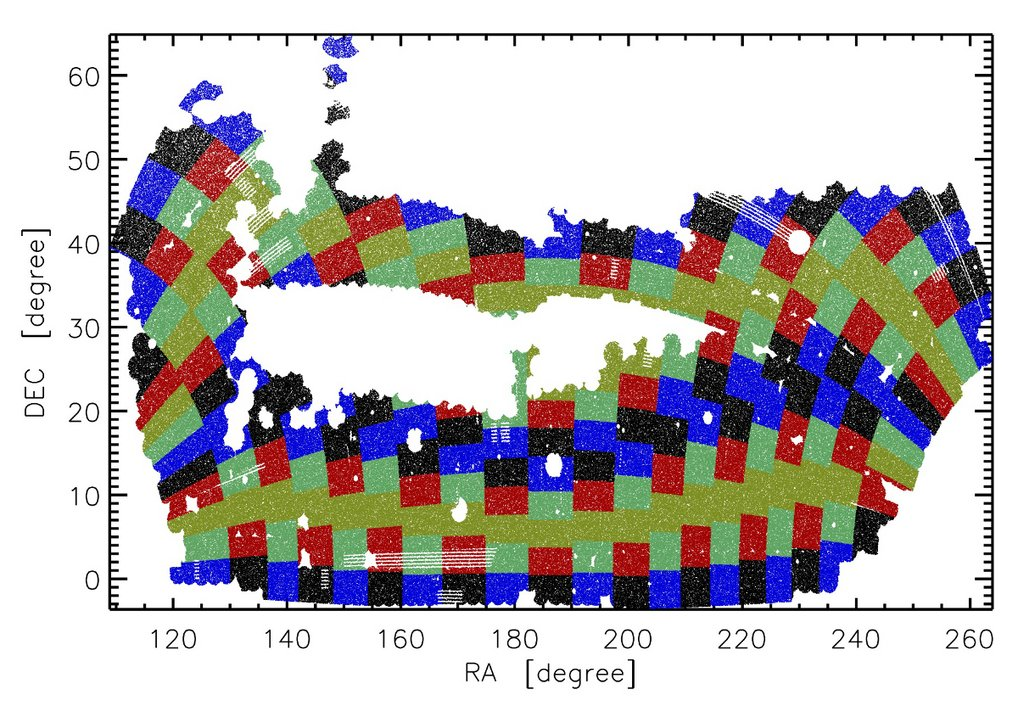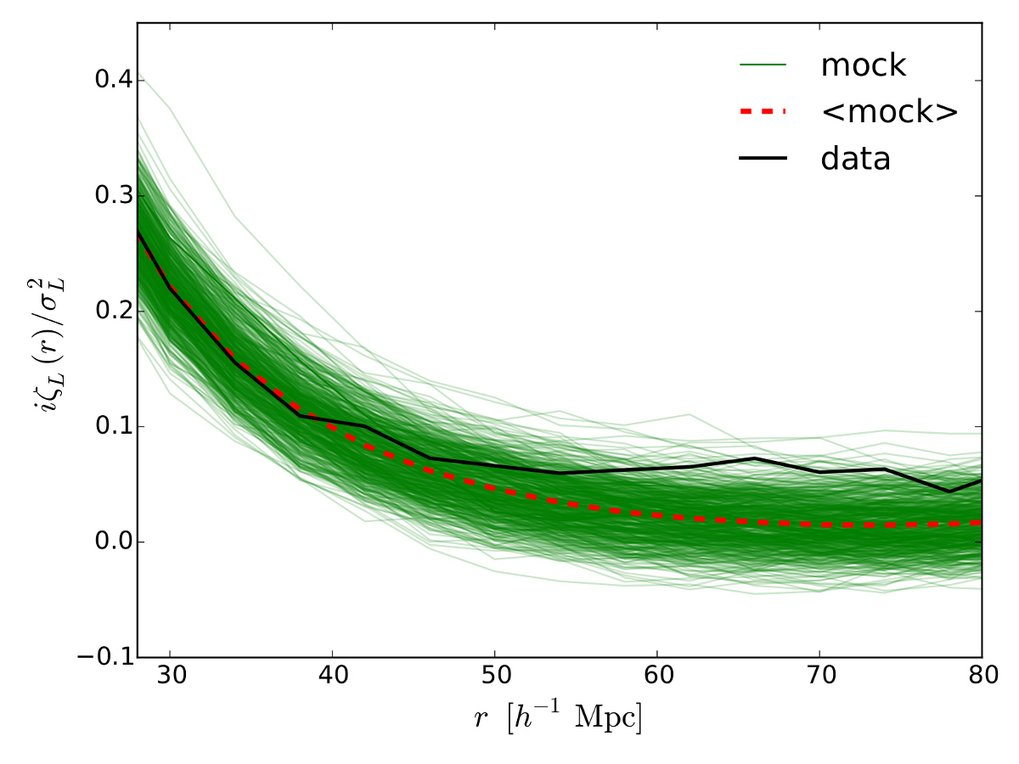# A new observable of the large-scale structure: the position-dependent two-point correlation function

June 01, 2015

Observations of the large-scale structure, such as galaxy surveys, are one of the most important tools to study our universe. In particular, how the growth of structure is affected by the large-scale environment can be used to test our understanding of gravity, as well as the physics of inflation. A research group at MPA has recently developed a new technique to extract this signal more efficiently from real observations. Specifically, we divide a galaxy survey into sub-volumes, quantify the structure and the environment in each sub-volume, and measure the correlation between these two quantities. This technique thus opens a new avenue to critically test fundamental physics from real observations.Fig. 1: Projected slice of the galaxies observed in the SDSS with distances (redshifts). (The position on the sky is measured in observation coordinates: RA labelled in hours, with DEC being projected onto the plane.) The yellow, red, and white points are the main galaxy sample, the red luminous galaxies, and the BOSS CMASS sample, respectively. Credit:Michael Blanton and SDSS collaboration

The large-scale structure is one of the most important observables in modern astronomy to probe the properties of our universe. Large galaxy survey programmes such as the 2dF Galaxy Redshift Survey and the Sloan Digital Sky Survey (SDSS) measure the angular positions on the sky and the distance (redshift) of millions of galaxies, currently out to 6.4 billion years ago. Scientists then use these data to construct a three-dimensional map of our universe, as shown in figure 1.

As the visual rendering in figure 1 shows, one can clearly see filamentary structures as well as relatively empty regions. This is how our universe looks like. To quantify these structures of our universe, scientists use in particular the so-called "two-point correlation function," which measures how likely it is to find galaxies in pairs with some given separation. For example: if we choose a separation of 150 Mpc (which corresponds to 490 million light-years or 4.6 sextillion kilometres), we then count the number of galaxy pairs that we can find with a distance of 150 Mpc between them. Once we are done with this separation, we move on to the next separation we are interested in. As we keep doing this counting, we get the two-point correlation as a function of separation.Fig. 2: The two-point correlation function of the BOSS DR10 CMASS sample. The orange data points are the measurements for the observed galaxies, the dashed line denotes the expectation from the currently accepted cosmological model.  Credit: Ariel G. Sánchez and SDSS collaboration

The orange data points in figure 2 show the measurement of the two-point correlation function from the galaxies observed in the SDSS. At a separation of roughly 150 Mpc we find a small bump. This means that it is more likely to find galaxy pairs with this separation compared to smaller or larger distances. This bump was imprinted only 400,000 years after the Big Bang by sound waves in the plasma filling the (then ionized) universe.

While the two-point correlation function is the most common statistic to quantify structures in our universe, the observed galaxies contain more information. One interesting question, in particular, is if and how the structures depend on their large-scale environment. More specifically, we want to study whether or not there will be more structure in a relatively over-dense region compared to an under-dense region.

This question can be addressed by the "three-point correlation function", i.e. looking for three galaxies with given separations. However, these measurements rely on finding galaxy triplets, which is computationally challenging due to the large number of observed galaxies.Fig. 3: The division of the BOSS DR10 CMASS sample into sub-volumes on the sky. Each coloured block extends over the whole redshift range. © MPA

Recently, a research group at MPA has developed a new method, the position-dependent two-point correlation function, to address the question of how structure depends on the environment and capture this particular signal from the observed galaxies. Specifically, for a given galaxy survey, we divide the entire survey volume into small sub-volumes (see Figure 3). We then measure the mean over-density (with respect to the entire survey) and the two-point correlation function in each sub-volume, to get a position-dependent two-point correlation function. Finally, we measure the correlation between these two quantities. If we find a positive correlation, this means that it is more likely to find more structures in the over-dense background, and vice versa. In mathematical terms, this correlation measures an integral over the three-point function; therefore we call it the integrated three-point function. Since this new method requires only the counting of galaxy pairs, the computational problems with the three-point function are largely alleviated.Fig. 4: First measurement of the integrated three-point function from the BOSS DR10 CMASS sample. The thick black solid line shows the measurement for real data, the thin green lines show the results for each of the 600 mock realisations, while the thick red dashed line shows the mean of the mock realisations. © MPA

We apply this new technique to "real" and "mock" data of a sample of SDSS galaxies, the BOSS DR10 CMASS sample. The real data contain positions and distances (redshifts) for about 0.4 million observed galaxies, while the 600 mock catalogues were generated by simulations to match the properties of the real data for data analysis. The first measurement of the integrated three-point function for the BOSS DR10 CMASS sample is shown in figure 4. We find that even though the integrated three-point function of the observed galaxies does not agree perfectly with the mean of the mock realisations, it is within the scatter of the simulated results. Moreover, both the measurements for the real data and the mean mock results are above zero for all separations, meaning that in our universe the structures do grow more strongly if they are in an over-dense environment.

The coupling between small-scale structures and their environment or background plays a fundamental role in cosmology. This correlation arises because of gravitational evolution, and possibly from inflationary physics. This new observable, the position-dependent two-point correlation function, therefore allows us to test our understanding of gravity and the physics of inflation. Combining our first measurement with other probes such as the global two-point correlation function and the weak lensing signal, we are able to constrain how galaxies trace the underlying dark matter density. In the future, with better data, we shall utilise this technique to study the properties of inflation, which is one of the biggest mysteries in physics and at the same time provided the seeds for all present-day structures.

Chi-Ting Chiang

## Other Interesting Articles

Go to Editor View